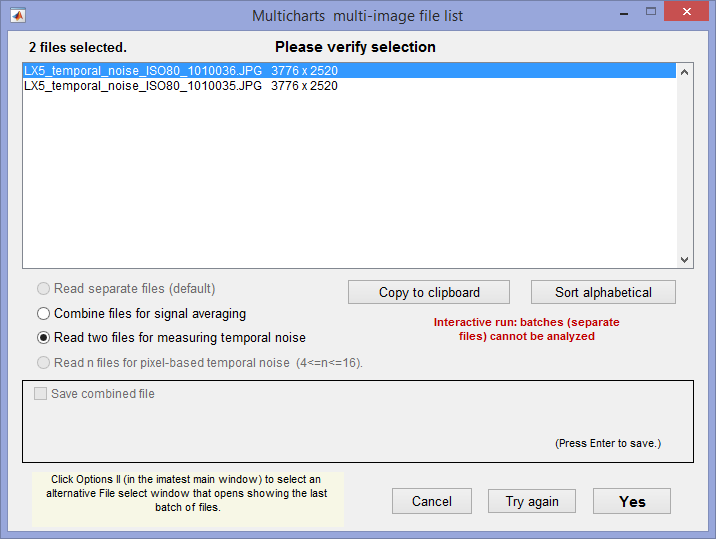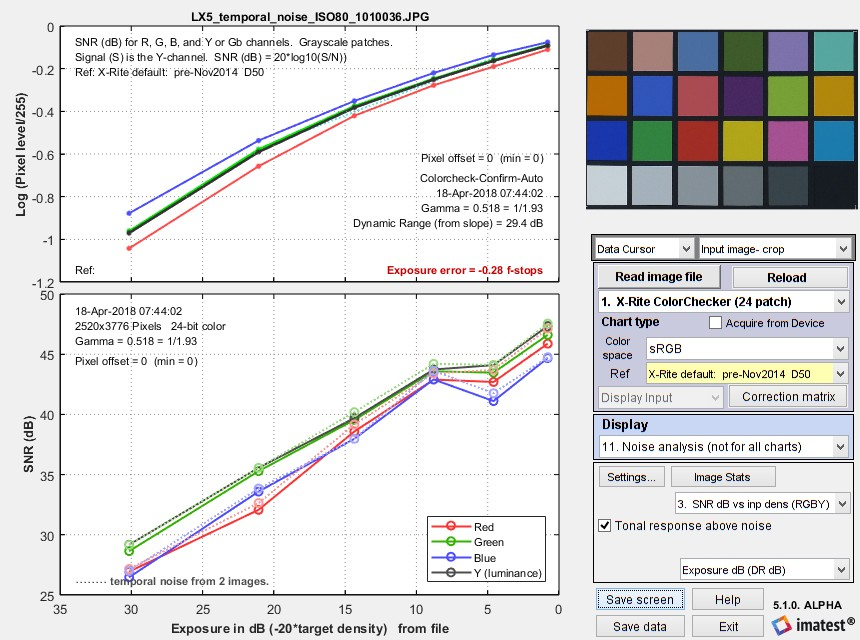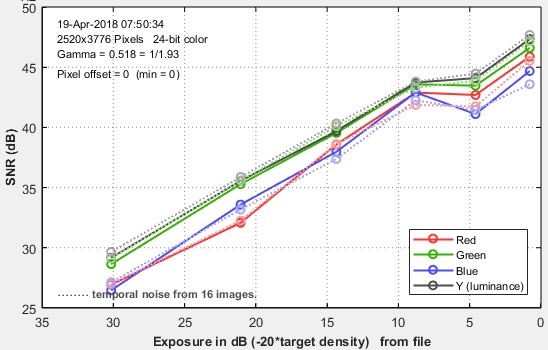# Measuring temporal noise

April 19, 2018
April 21, 2018

Temporal noise is random noise that varies independently from image to image, in contrast to fixed-pattern noise, which remains consistent (but may be difficult to measure because it is usually much weaker than temporal noise). It can be analyzed by Colorcheck and Stepchart and was added to Multicharts and Multitest in Imatest 5.1.

It can be calculated by two methods.

1. the difference between two identical test chart images (the Imatest recommended method), and

2. the ISO 15739-based method, which where it is calculated from the pixel difference between the average of N identical images (N ≥ 8) and each individual image.

In this post we compare the two methods and show why method 1 is preferred.

(1) Two file difference method. In any of the modules, read two images. The window shown on the right appears. Select the Read two files for measuring temporal noise radio button.

The two files will be read and their difference (which cancels fixed pattern noise) is taken. Since these images are independent, noise powers add. For indendent images I1 and I2, temporal noise is

$$\displaystyle \sigma_{temporal} = \frac{\sigma(I_1 – I_2)}{\sqrt{2}}$$

In Multicharts and Multitest temporal noise is displayed as dotted lines in Noise analysis plots 1-3 (simple noise, S/N, and SNR (dB)).

(2) Multiple file method. From ISO 15739, sections 6.2.4, 6.2.5, and Appendix A.1.4. Available in  Multicharts and Multitest. Currently we are using simple noise (not yet scene-referred noise). Select between 4 and 16 files. In the multi-image file list window (shown above) select Read n files for temporal noise. Temporal noise is calculated for each pixel j using

$$\displaystyle \sigma_{diff}(j) = \sqrt{ \frac{1}{N} \sum_{i=1}^N (X_{j,i} – X_{AVG,j})^2} = \sqrt{ \frac{1}{N} \sum_{i=1}^N X_{j,i}^2 – \left(\frac{1}{N} \sum_{i=1}^N X_{j,i}\right)^2 }$$

The latter expression is used in the actual calculation since only two arrays, $$\sum X_{j,i} \text{ and } \sum X_{j,i}^2$$, need to be saved. Since N is a relatively small number (between 4 and 16, with 8 recommended), it must be corrected using formulas for sample standard deviation from Identities and mathematical properties in the Wikipedia standard deviation page as well as Equation (13) from ISO 15739.  $$s(X) = \sqrt{\frac{N}{N-1}} \sqrt{E[(X – E(X))^2]}$$.

$$\sigma_{temporal} = \sigma_{diff} \sqrt{\frac{N}{N-1}}$$

We currently recommend the difference method (1) because our experience so far has shown no advantage to method (2), which requires many more images (≥ 8 recommended), but allows fixed pattern noise to be calculated at the same time.

To calculate temporal noise with either method, read the appropriate number of files (2 or 4-16) then push the appropriate radio button on the multi-image settings box.Multi-image settings window, showing setting for method 1.
if 4-16 images are enterred, the setting for method 2 (Read n files…) will be available.

### Results for the two methods

The two methods were compared using identical Colorchecker images taken on a Panasonic Lumix LX5 camera (a moderately high quality small-sensor camera now several years old).

#### Difference method (1) (two files)

Here are the Multicharts results for 2 files.Multicharts SNR results for temporal noise, shown as thin dotted lines in the lower plot

#### Multi-file method (2) (4-16 files)

 Here are results (SNR (dB)) for runs with 4, 8, and 16 files. For 4 files, temporal SNR (thin dotted lines) is slightly better than standard noise. Temporal SNR is slightly lower for 8 files and very slightly lower for 16 files. For 8 and 16 files results are closer to the results for 2 files (though differences between 8 and 16 files are very small). The bottom line: We recommend the two-file (difference) method because it is accurate and relatively fast. The multi file method is slower for acquiring and analyzing images— at least 8 images are recommended, so why bother?Temporal SNR from 4 imagesTemporal SNR from 8 imagesTempoal SNR from 16 images

This site uses Akismet to reduce spam. Learn how your comment data is processed.